Directions: Fill in the blanks with integers so that the quadratic expression is factorable.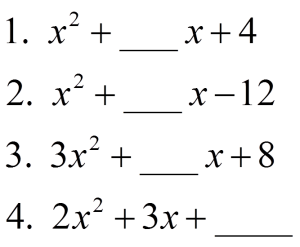### Hint

Give me a number that you know does not work.  How can you prove that it does not work?  How can you use this information to find a number that does work?

1. 5, -5, 4, -4
2. 11, -11, 4, -4, 1, -1
3. 25, -25, 14, -14, 11, -11, 10, -10
4. 1, -5, -2, -9, …this goes on and on.  Can you find a pattern?

Use Algebra tiles to see this physically.  I found it to be easier if I used Algebra Tiles, especially with the last problem, which, by the way, has more than a couple answers.

By the way, I usually teach factoring with Algebra Tiles.

Here is how I set up Algebra Tiles.  When you use tiles to factor, you are trying to arrrange the tiles into a rectangle (think area model).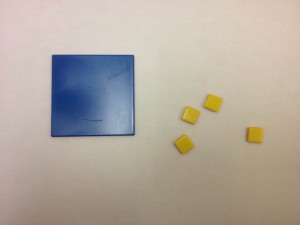First, I grab the necessary tiles.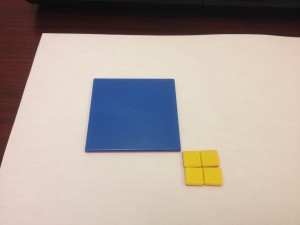Then, I arrange the yellow tiles into a rectangle.

All (big blue square and rectangle of yellow small unit squares) of the pieces will make a rectangle, with missing chunks, the missing chunks represent the missing b term in the quadratic.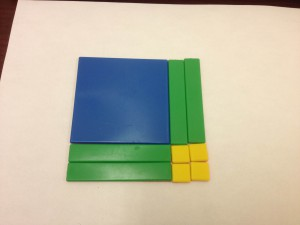Then, fill in the missing chunks with the skinny (green, means positive) rectangles, representing x.

Then, fill in the missing chunks with the skinny (red, means negative) rectangles, representing x.

You can now go back to the first picture and see if there are any more possible rectangles that can be arranged with the yellow pieces.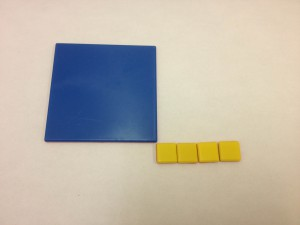By the way, this get trickier if the pieces are negative.  Enjoy!

Source: Nanette Johnson

## Difference of Squares and Sum of Cubes

Directions: Using the digits 0 to 9 at most one time each, place a digit …

1.1. x² + 4x + 4
2. x² + 4x – 12
3. 3x² + 14x + 8
4. 2x² + 3x – 2

2.1. 5
2. -11
3. 25
4. -2